# Splines in Onshape, part 2

In part 1, we talked about 2D splines and how they can be created in Onshape sketches. In this second part, I’ll briefly cover drawing splines directly in 3D, and then discuss offset curves, which are the original reason I started on this long journey delving into Onshape curves.

### Directly creating 3D splines in Onshape

There are two FeatureScript functions that can be used for creating splines directly in 3D: opFitSpline() and opCreateBSplineCurve().

opFitSpline() is exactly equivalent to the skFitSpline() function described earlier, except instead of working in the context of a 2D sketch (with an implicit sketch plane), it directly draws a curve in 3D. This can be more convenient than going via a sketch, and the curve need not be constrained to a single plane. The parameters are exactly the same as skFitSpline(), except that all of the points are specified as 3D instead of 2D vectors.

opCreateBSplineCurve() is the most powerful of the spline functions, and also the most daunting. It takes a set of B-spline knots and control points (refer back to part 1 for a crash course on B-splines). Unlike the other functions, this function lets you create splines of arbitrary degree, not just cubic splines. It also allows you to create closed splines (more on this shortly), and it allows you to create rational splines aka NURBS. I won’t go into rational splines in this article, but they allow you to draw certain curves that wouldn’t otherwise be possible to express in B-spline form.

To demonstrate use of these functions, here’s a 3D object that I’ve created by drawing a closed cross-sectional profile with opCreateBSplineCurve() and a guide path with opFitSpline(). I then swept the profile along the guide path with opSweep() and thickened the resulting surface with opThicken() to create a 3D object.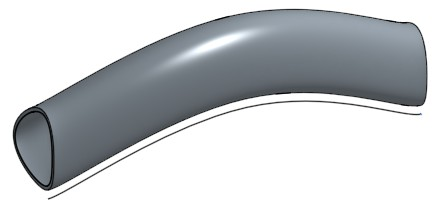(I’ve left the guide path in the picture for illustration purposes.)

I’m not sure what this object actually is, perhaps it’s a handle for something, or an art piece for my future Onshape museum. But you can see how this might be useful if we need to create a computed surface in an actual engineering problem. Here is the full code:

// cross-sectional profile (closed spline in YZ plane)
// explanation follows after code
var degree = 3;
var isClosed = true;
var cpts = [vector(0,0,0)*meter, vector(0,-10,30)*meter,
vector(0,30,10)*meter, vector(0,10,0)*meter];
// repeat first 3 (degree) control points at end
cpts = concatenateArrays([cpts,resize(cpts,degree)]);
var knots = range(0,1,len(cpts)+degree+1);
var bspline = bSplineCurve(degree, isClosed, cpts, knots);
opCreateBSplineCurve(context, id + "spline1", {
"bSplineCurve" : bspline
});

// path for sweep (interpolated spline in XY plane)
opFitSpline(context, id + "spline2", {
"points" : [
vector(   0,  0, 0) * meter,
vector(  50, 20, 0) * meter,
vector( 100,  0, 0) * meter
],
"startDerivative" : vector( 30, 0, 0 ) * meter,
"endDerivative" :   vector( 30, 0, 0 ) * meter
});

// sweep spline1 along spline2
opSweep(context, id + "sweep", {
"profiles" : qCreatedBy(id + "spline1", EntityType.EDGE),
"path" : qCreatedBy(id + "spline2", EntityType.EDGE),
"keepProfileOrientation" : true
});

// thicken to create solid body
opThicken(context, id + "thicken", {
"entities" : qCreatedBy(id + "sweep", EntityType.BODY),
"thickness1" : 0 * meter,
"thickness2" : 1 * meter
});

// normally we'd delete the sweep path
// opDeleteBodies(context, id + "deletespline2", {
//         "entities" : qCreatedBy(id + "spline2")
// });


One thing that warrants explanation is how I’ve selected the B-spline parameters to create a closed (‘periodic’) spline. For all of the B-splines discussed in part 1, I repeated the first and last knot four times to ensure that the spline passed through the start and end point (the knot sequences looked like [0,0,0,0,0.25,1,1,1,1], for example). In this case, we don’t need or want to clamp the curve to fixed endpoints. Instead, I’ve chosen a uniform set of knots between t=0 and t=1 ([0,0.1,0.2,…,0.8,0.9,1]). The resulting curve does not pass through the first or last control point, but is still guided by the control polygon. I then repeat the first three control points at the end of the list of control points — wrapping part of the control polygon around the curve a second time — which results in a closed spline.

Here is a plot of the spline and control polygon that might help illustrate this. The control polygon starts at the origin (0,0) and goes clockwise around through (-10,30), (30,10), (10,0) and back to (0,0), then retraces (-10,30), (30,10), (10,0). The resulting spline follows the left dotted line from the origin to the top of the egg (from t=0 to t=0.3), traces the egg once (from t=0.3 to t=0.7), and returns to the origin via the right dotted line (from t=0.7 to t=1). When Onshape draws the curve, it only draws the middle part from t=0.3 to t=0.7, so the result is the closed egg shape. (For a spline with degree 3, the outer three knot spans are degenerate — in that the basis does not add to 1 and the spline tends towards the origin regardless of control points — so these parts of the spline are always ignored when drawing.)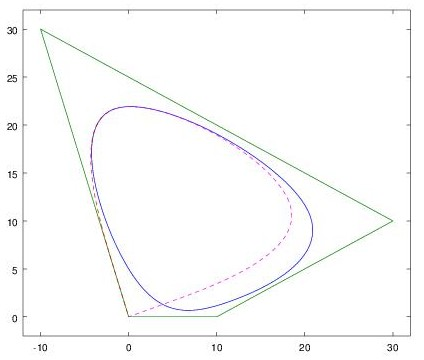### Offset curves

Sometimes it’s necessary to generate a curve that’s “parallel” to another curve, maintaining a constant distance from it. In CAD this is called an offset curve. Here is an example in which I’ve drawn a curve and an offset curve at a given distance. I’ve then used the two curves to create a curved object with constant thickness: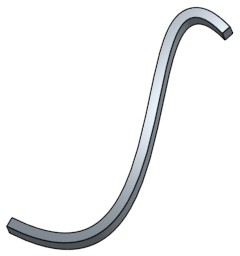Note that drawing an offset curve is not as simple as translating the curve (moving it in (x,y)). If I was to create this object by copying the bottom curve, dragging the copy towards the top left, and filling in the area between the two, I end up with something that is far from constant thickness: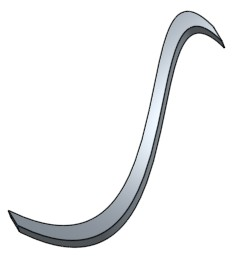Rather, to create the offset curve, each point on the curve needs to be moved in a slightly different direction: the direction normal to the curve at that point.

The Onshape sketch GUI has an “offset” tool which makes offsetting a curve easy. I needed to do this programmatically in FeatureScript, however, and there are no documented FeatureScript functions for offset curves. I tried a number of approaches ranging from mathematics to advanced Onshape-wrangling. If you’re just interested in how to do it, you can skip down a few sections to the code.

### Calculating offset curves, from first principles

Let’s start from the simplest case, a 2D spline with one piece (a 2D Bézier curve). This is a simple polynomial which, as mentioned in part 1, we can write in the parametric form:

(1)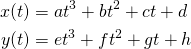To produce the offset curve, each point on the curve needs to be translated by a constant distance (call it D) in a direction normal to the curve (n̂(t)). Thus we can write the offset curve as: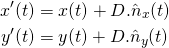The unit normal n̂(t) is just the unit tangent rotated by 90 degrees. This 90 degree rotation is trivial; it is simply a co-ordinate rotation where (x,y) becomes (-y,x). (If we represent (x,y) in complex form as as x+iy we simply multiply by i, which results in ix+i2y = ix-y.). So we can rewrite the above equations in terms of the unit tangent: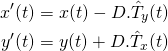The tangent vector to a curve can be evaluated as (dx/dt, dy/dt), or in more convenient notation (ẋ(t),ẏ(t)), where a dot above a variable represents a derivative with respect to t. However, we need to scale the tangent to a unit vector by dividing by its length, sqrt(ẋ(t)2+ẏ(t)2). Substituting:

(2)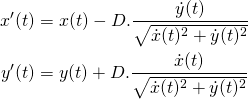Now you can see where things are starting to get ugly. We can’t avoid the square root term in the denominator; D must multiply a unit vector or the new curve wouldn’t be a fixed distance away. In order to eliminate the square root in the denominator and turn this back into a polynomial, we have to rearrange and square… but then we no longer have x'(t) and y'(t) alone on one side.

It turns out that it’s not actually possible to express the offset curve in parametric polynomial form x'(t)=f(t) and y'(t)=g(t), regardless of the degree of f and g. Perusing the literature on the subject, we find that it’s theoretically possible to express the offset curve as a 10th order implicit polynomial, in the form f(x,y)=0 for some terrifying array of terms . But this is about as useful to us as tits on a bull.

Because we can’t express the offset curve in parametric polynomial form, we can’t input it directly into Onshape as a spline. We can, however, use the above equations to evaluate the offset curve numerically at any point… assuming that we can easily evaluate ẋ(t) and ẏ(t).

### Side note: derivatives of splines

If x(t) and y(t) are in polynomial form as in equation set 1, the first derivatives ẋ(t) and ẏ(t) follow trivially using the standard method of differentiating polynomials (ẋ(t) = 3at2 + 2bt + c, etc.). If x(t) and y(t) are in B-spline form, it turns out that there is also a simple method to generate the first derivative.

The derivative of a B-spline is a B-spline of one degree less, with the outer knots removed (e.g. a degree 3 spline with knots [0,0,0,0,0.25,1,1,1,1] becomes a degree 2 spline with knots [0,0,0,0.25,1,1,1]). The control points are replaced with the pairwise differences of control points (P2−P1, P3−P2, etc.), with some scaling corrections based on the knot distances. I’ve implemented this algorithm in the Octave/MATLAB function bsplinederiv.m.

### Evaluating offset curves, numerically

Now we can easily evaluate the offset curve for a B-spline using the above equations. Here I’ve plotted the spline from the figures above, and its offset curve, numerically in Octave:

octave:1> degree = 3;
octave:2> cpts = [0+0i;100-50i;50+150i;100+100i];
octave:3> knots = [0,0,0,0,1,1,1,1];
octave:4> [dcpts,dknots,ddegree] = bsplinederiv(degree,cpts,knots);
octave:5> t = [0:0.01:1];
octave:6> offset = 5;
octave:7> spline = bsplineeval(degree,cpts,knots,t);
octave:8> splinederiv = bsplineeval(ddegree,dcpts,dknots,t);
octave:9> unitnormal = normalize(splinederiv*i);
octave:10> plot(spline,'-',spline+offset*unitnormal)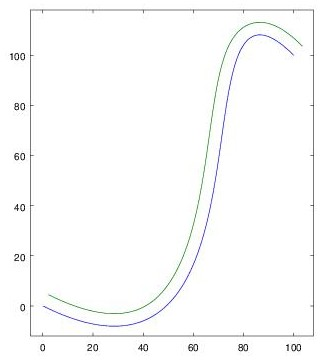In theory we could import this into Onshape as a polyline approximation to the curve (made up of many line segments). With enough line segments, one could make the approximation ‘good enough’ for any given purpose. But this doesn’t seem very satisfying; it would be nice to have an actual curve at the Onshape kernel level so that there’s no risk of artefacts.

### Approximations to offset curves

While we’ve found that the exact offset spline can’t be written as a parametric polynomial, another approach would be to find a polynomial that’s ‘close’ to it. There are many different ways of doing this. The method I tried was to start with two assumptions:

1. The offset spline should start/end at points that are correctly offset from the start/end points of the original spline.
2. The tangent to the offset spline should be parallel to the tangent of the original spline at these end points.Neither of these requirements on the end points are strictly required; if we were to relax them we might be able to come up with curves that are a better approximation at other points. But constraints on the end points are convenient because they will make it possible to offset a spline piece-by-piece and have the offset splines match up.

For a simple one-piece spline, aka a Bézier curve, this only leaves two parameters unspecified: the scale values α and β on the start and end derivatives.

In order to lock down α,β let’s arbitrarily pick two more points along the offset curve and solve for the required α,β so the curve passes through these points: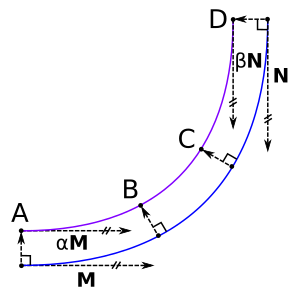It may seem counter-intuitive that we can fit a spline through four points and two tangent directions given that we only have eight free parameters in our parametric polynomial. Frankly, this had me scratching my head for a while too. But — referring back to the parametric polynomial defined in equation set 1 — note that when we specify an (x,y) without a t value, we’re providing (x,y) but introducing a new unknown for t, so one fit point only reduces the equation set by one unknown.

After a little algebra this produces the following equation set, where t1 and t2 are the unknown t values corresponding to points B and C on the offset curve, α and β are the unknown scale values for the derivatives M and N, and everything else is a known constant defined in terms of point locations (P = BA; Q = CA; R = DA).Perhaps due to masochism, I had a burning desire to try and solve this algebraically, i.e. to try and find a closed form solution for the variables α,β in terms of known quantities. After all, it seems like a relatively simple geometric problem, and we have four equations and four unknowns.

A naïve approach might be to use the general cubic solution to rearrange equations (3) and (4) for t1, and set the two expressions equal, eliminating t1. Repeat for (5) and (6) to eliminate t2, and finally solve for α and β. This should work in theory, but if you’ve ever tried to use the general cubic solution, you’ll know why this is problematic. It soon blows up into tens of terms, resulting in many pages of working and much loose hair.

I also tried entering this equation set into a couple of symbolic computation packages (Maple and SAGE) and praying for a solution (solve()). In both cases they ran out of memory without a result. The reason is that they attempt to apply a general method for solving simultaneous polynomial equations, based on computing Gröbner bases (click if you dare, but honestly that Wikipedia page is pretty impenetrable to anyone who doesn’t already have a PhD in computing Gröbner bases). The method is exponential in the number of variables, and while it can handle large polynomials over a handful of variables, it is not a good method when there are many variables.

I threw down the gauntlet on Facebook, calling on maths geeks everywhere to help. To my disappointment, no-one tried to attack this algebraically; all of the suggestions leaned towards numerical approaches. One commenter (Kenneth Tsui) very kindly sent me an Excel spreadsheet that implemented the solution numerically using Newton’s method, an iterative method for successively improving an initial guess by gradient descent of the error function. The geometry of the problem is such that we can make good initial guesses (α = 1, β = 1, t1 = 1/3, t2 = 2/3), so this method actually works very reliably and only takes three iterations to converge: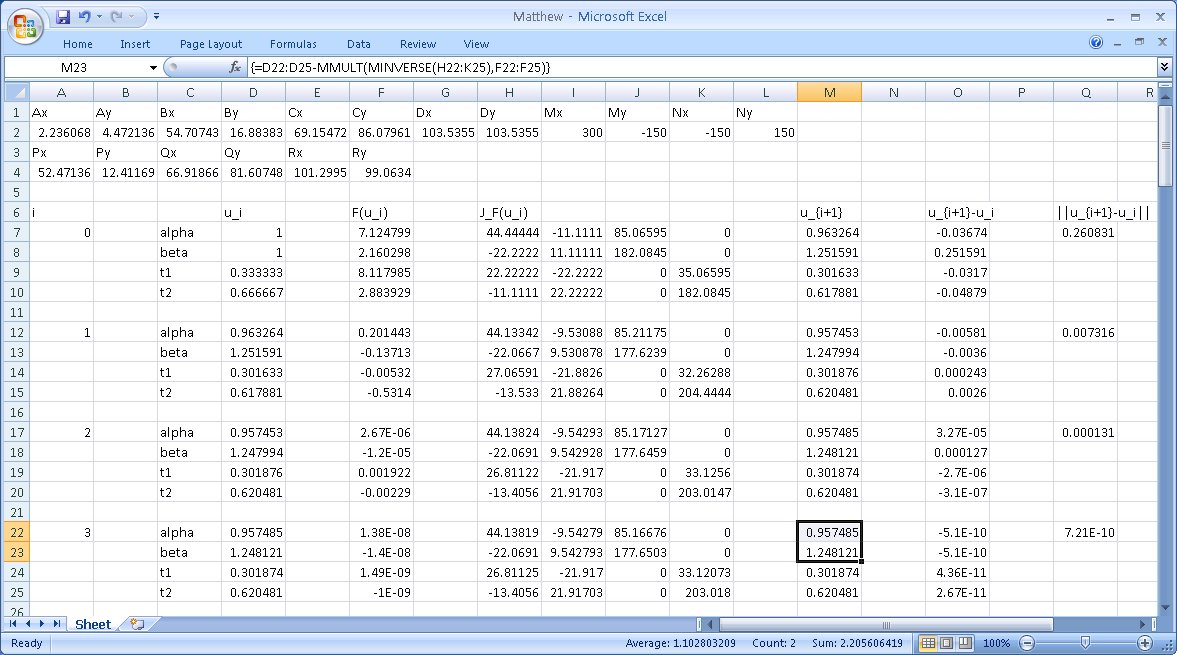Personally I would never have thought to use Excel like this, but it turns out that even a hatchet can be used to hammer in a nail 😉

I tried this for a few splines. For simple splines the results are good, but when there’s high curvature the deviation can be noticeable (green is the real offset curve, red the approximation):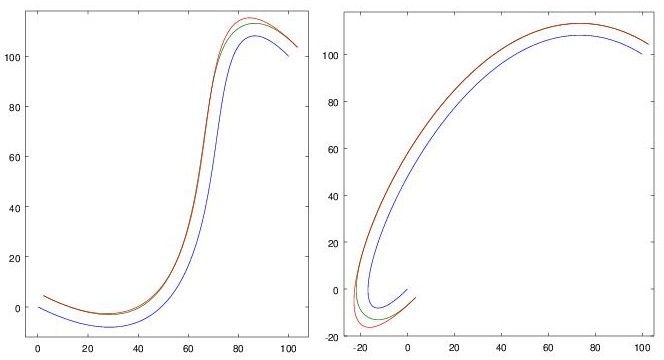One could improve the result with a more targeted choice of points B and C, or by adding more freedom to the offset spline — perhaps a higher order polynomial, or more control points — but the mathematics was breaking my head already just trying to fit a cubic Bézier. Let’s go back a step and try another approach…

### Offset curves, using Onshape constraints

Remember that I mentioned that Onshape is able to create an offset curve quite effortlessly when using the offset tool in the sketch GUI. How does it do this?

Onshape has a handy feature that you can view the code generated by the GUI (right click on a Part Studio → View Code). By perusing the code produced by the offset tool, I did eventually figure out how to create an offset curve from FeatureScript. The key is to create a new spline using skSpline() or skSplineSegment(), and then add a DISTANCE constraint that constrains it to be a certain distance away from the first spline:

skFitSpline(sketch, "spline1", {
"points" : [
vector(0,0) * meter,
vector(100,100) * meter
],
"startDerivative" : vector(300,-150) * meter,
"endDerivative" : vector(150,-150) * meter
});
skSplineSegment(sketch, "spline2", {});
skConstraint(sketch, "distance", {
"constraintType" : ConstraintType.DISTANCE,
"localFirst" : "spline1",
"localSecond" : "spline2",
"length" : 5 * meter,
"direction" : DimensionDirection.MINIMUM,
"alignment" : DimensionAlignment.ANTI_ALIGNED
});
// not strictly required?
skConstraint(sketch, "offset", {
"constraintType" : ConstraintType.OFFSET,
"localMaster" : "spline1",
"localOffset" : "spline2"
});


The OFFSET constraint does not seem to be required for the solver to do the right thing here, it may just be for GUI display purposes, but I’ve left it in the code example just in case.

There is one oddity with this. We have not specified any constraints on the endpoints, and sometimes the solver takes more liberty with the second endpoint than you might like. For example, depending on spline parameters, you can get something like this:I’ve not been able to figure out the logic of how the solver chooses the second endpoint, however this is of purely academic interest — in practice, the requirements of the CAD problem will usually dictate physical constraints on the endpoints. If you want to force the second curve to end at the same point as the first curve, you can add an extra constraint between the endpoints, for example:

skConstraint(sketch, "distance.end", {
"constraintType" : ConstraintType.DISTANCE,
"localFirst" : "spline1.end",
"localSecond" : "spline2.end",
"length" : 5 * meter,
"direction" : DimensionDirection.MINIMUM,
"alignment" : DimensionAlignment.ANTI_ALIGNED
});


In the earlier picture where I used an offset spline to create a 3D object, I needed to join the spline ends with two line segments to form a face. As in the sketch GUI, this can be done by using COINCIDENT constraints. (The code is simple but rather verbose; if you need many such constraints in your FeatureScript it may be worth writing a makeCoincident() function.)

skLineSegment(sketch, "line1", {});
skConstraint(sketch, "coincident.line1.start", {
"constraintType" : ConstraintType.COINCIDENT,
"localFirst" : "line1.start",
"localSecond" : "spline1.start"
});
skConstraint(sketch, "coincident.line1.end", {
"constraintType" : ConstraintType.COINCIDENT,
"localFirst" : "line1.end",
"localSecond" : "spline2.start"
});
skLineSegment(sketch, "line2", {});
skConstraint(sketch, "coincident.line2.start", {
"constraintType" : ConstraintType.COINCIDENT,
"localFirst" : "line2.start",
"localSecond" : "spline1.end"
});
skConstraint(sketch, "coincident.line2.end", {
"constraintType" : ConstraintType.COINCIDENT,
"localFirst" : "line2.end",
"localSecond" : "spline2.end"
});


The sketch GUI also has a “slot” tool that allows you to conveniently draw two offset curves, with semicircular end caps. This can be implemented in FeatureScript following the same approach as the above code, replacing skLineSegment() with skArc(); indeed under the bonnet this is what the slot tool generates.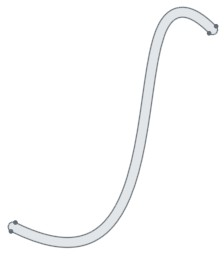### Offset curves in Onshape

While we can now create an offset curve in a sketch, we still haven’t really answered the question of how Onshape is doing this magic. Have the wizards at Onshape cracked the problem of how to derive offset spline parameters from the original spline?

While their wizardry is impressive, the answer is no, Onshape actually uses a much simpler method. It stores an offset curve as the original spline parameters and an offset value. The points of the offset curve can then be calculated numerically at evaluation time using an approach equivalent to equation set 2. If you’ve attempted to follow my mathematics above, you’ll now appreciate how much simpler this is.

Unfortunately, there does not seem to be any way (that I can find) to specify the offset value explicitly when creating a spline. For splines used in sketches, you can use a DISTANCE constraint as shown above, and the solver will assign the right offset to the second spline. This is a little tedious but works well. However it can’t be applied to splines created outside of sketches (opFitSpline() or opCreateBSplineCurve()); in this case there is no way that I can find to specify the offset value. I hope that the ability to specify an explicit offset might be added in a future version.

### Side note on Onshape rendering

As a side note, while the Onshape computational engine evaluates curve points precisely where needed, the objects displayed in the GUI are built using straight edges. The rendering is not refined as you zoom in. Thus, attempting to visually place elements may produce unexpected results. For example, below is a line segment that is constrained to be co-incident with an spline; in the zoomed-in GUI, there is a gap you could drive a large bacterium through. The line segment does, however, touch the real spline.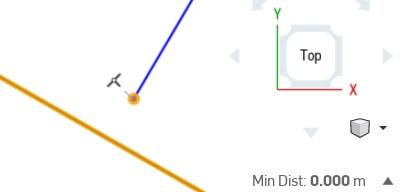For the most part the accuracy of the GUI approximation is a moot point of course. The underlying solver does evaluate the points precisely, and the generated geometry is correct. The take home message is that — as in any parametric CAD system — you should specify any constraints that you need and not rely on visual placement in the GUI.

### Offset curve self-intersection

When drawing offset curves on the concave side of a spline, there is a problem that sometimes arises: the offset curve may run into itself (in fact, this always happens for some offset distance, but it’s most problematic when the spline has high curvature). If we blindly apply equation set 2 and plot the curve that results, we see that the offset curve has a loop: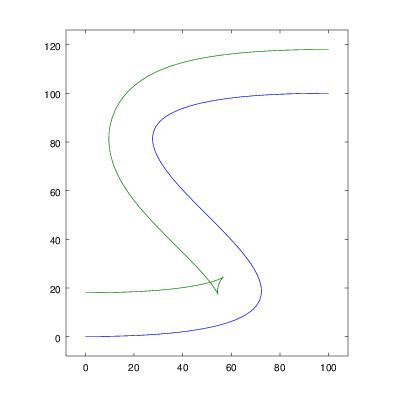(If you’re surprised by the shape of this loop, take something like an eraser and sweep it along the path; there is a point where the top of the eraser has to start moving downwards to make it around the tight bend.)

In this case, Onshape throws up its hands and refuses to create the offset curve. Sometimes this can happen even when one is not explicitly working with offset curves: for instance, the “thicken” operation I used earlier in this article requires the calculation of offset curves, which may fail depending on the thickness value. In that example, a value of 1 meter works okay, but 2 meters fails (in either direction), and it’s not immediately obvious where in the curve the self-intersection is. This can be quite frustrating when working with parametric objects; a small tweak in parameters can cause the object to suddenly fail to render.

There do exist algorithms that can trim loops from offset curves. Even after trimming the loop, there is still the complication that there is a cusp (vertex) in the curve where the loop was, which changes the geometry of any objects built from this curve: for example, if you were to extrude the above curve, there is now an extra edge in the knee. I’ve noticed that Onshape refuses to draw splines with cusps, which is likely to avoid this problem of surplus geometry. One way around this would be to interpolate an arc at the cusp (Maya can do this, for example), but this clearly involves a lot of added complexity in the computation.

I do hope that Onshape considers supporting some form of offset curve trimming in the future, or at least giving more detailed feedback when curve problems occur. This would make the system considerably most robust. As it is, I’ve found myself ‘testing’ my curves in Octave/MATLAB before throwing them at Onshape, otherwise I can end up with a blank sheet and no idea where I went wrong.

### Wrapping up

There’s a lot I haven’t covered in this article. In order to understand how Onshape represents offset curves, I sniffed the Websocket communication between the browser and the backend, which was an interesting rabbit hole. While the Developer Tools in both Chrome and Firefox can display Websocket communication, neither could dump binary Websocket streams in any useful format that I could use for further dissection. So instead I used mitmproxy to interpose on the SSL session and Wireshark/tshark to capture the communication. I then ran into the further obstacle that the Websocket traffic was compressed, and the Internet-given dissector plugin for this was deficient in a number of ways. A quick crash course in Lua later, and I have an improved version of this plugin on my github account (websocket_deflate_postdissector).

As usual I’ve learnt a lot of things about a lot of things in the process, and hopefully some of this information will be useful to you too. However, it’s probably time to get back to my actual design project.

### Postscript

I later realised a better way to tackle equations (3)–(7) algebraically might be the method of resultants; this allows eliminating t1 from equations (3) and (4) without having to rearrange for t1. (The resultant is sometimes known as an eliminant for this reason.) However, even with the method of resultants the closed form solution seems intractable. Here is a small sample of the polynomial equation I ended up with when solving for α: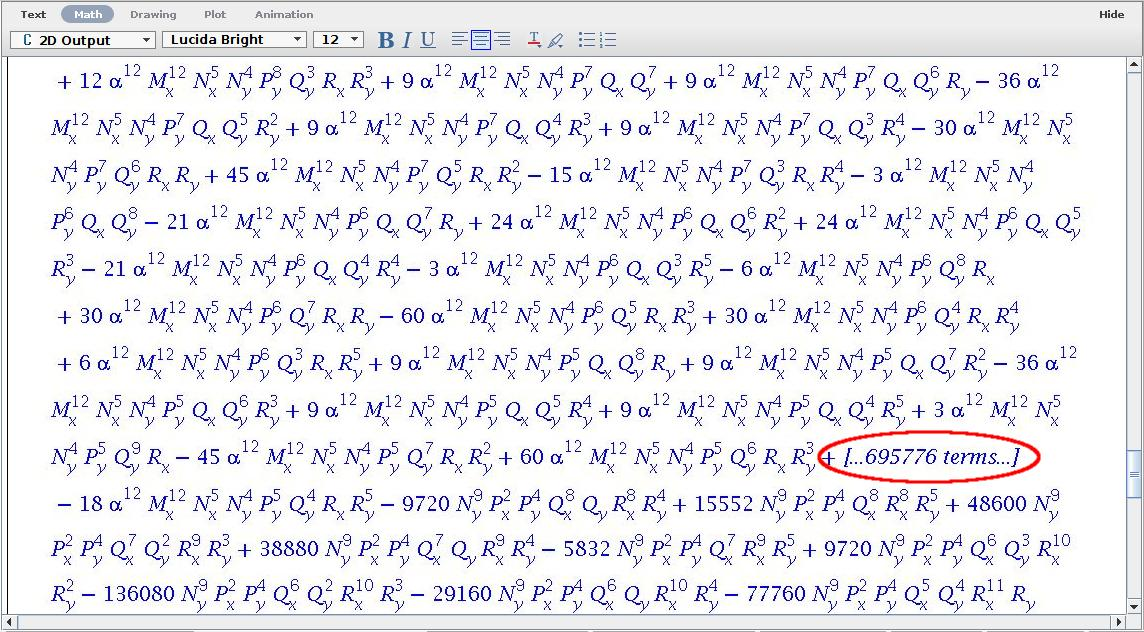Perhaps numerical methods are not so bad, after all.

This entry was posted in Computing. Bookmark the permalink.

### 5 Responses to Splines in Onshape, part 2

1.Bruce Bartlett says:

Great work Matthew, most went over my head but glad you’re enjoying Onshape. I am hoping we might be able to do an Onshape event in Sydney next year, maybe you can come and talk there. Also good discounts on the table ATM for pro plans to build numbers here in Australia, exciting times for CAD. Bruce

•Matthew says:

Thanks Bruce. Would love to see an Onshape event in Sydney.

2.Michał Lach says:

Hi, Matthew, love your work.
Did you try to investigate how Onshape eventually approximate spline offset? If you would extrude spline offset, I see no other way than to create a new spline.
I think that devs decided to do the “magic” to keep sketch solver more robust, but they must have some method to approximate new spline.

•Matthew says:

Thanks Michał. In theory I think it would be possible to keep the spline in the {spline,offset} form even if you extrude it – e.g. for a simple perpendicular extrude, the new edge becomes {spline+height,offset} with the same offset. I did some brief experiments and I think it may actually be done this way, there is no numerical error introduced by a simple perpendicular extrude. (I haven’t checked the network protocol though, that’s more effort to snoop.) However if I add a draft angle, either in the extrude or after, it does introduce some numerical error from the theoretical solution. From experience with other complex features in Onshape I would guess that this is implemented by evaluating points numerically and creating a new spline with many control points – probably subdividing until the error goes below a threshold – rather than trying to fit a spline with a fixed number of control points. I haven’t looked into it in detail though.

•Michał Lach says:

Thanks Matthew, I was just wondering if you had any chance to dig into this subject.